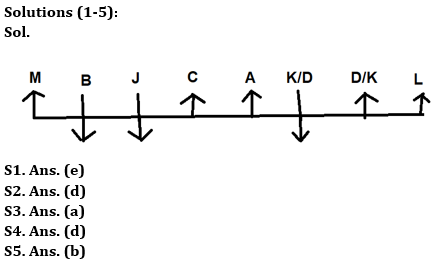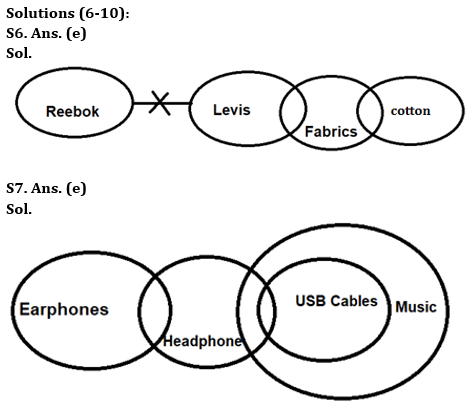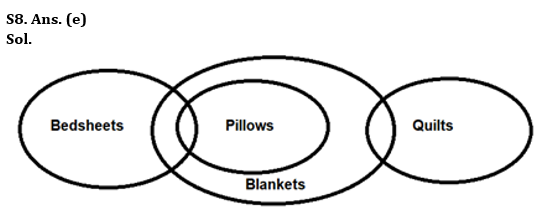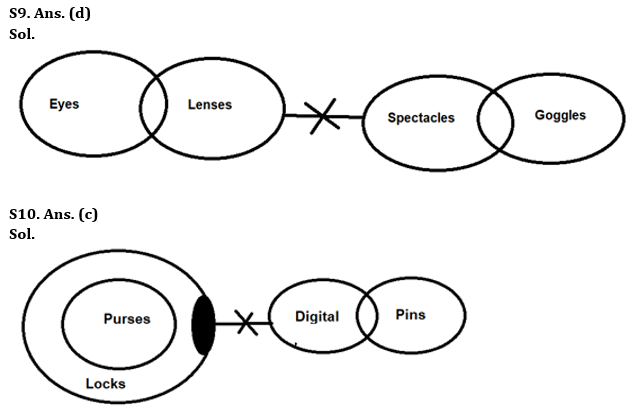Latest Banking jobs   »

# Reasoning Quizzes Quiz For IRDA AM 2023-08th May

Directions (1-5): Study the following information carefully and answer the given questions.
Eight persons, A, B, C, D, J, K, L and M are sitting in a row but not necessarily in the same order. Some of them are facing the north and others are facing south. B is sitting 3rd to the left of A and both of them are facing the opposite direction of each other. M who is sitting at one of the extreme ends, is sitting immediate right of B. C is sitting adjacent to A. L is sitting 4th to the right of C. Persons sitting at the extreme ends are facing the same direction as A. K and D are sitting immediate left of each other. K is not sitting to the right of J who is facing the south direction.

Q1. ____ is sitting adjacent to J.
(a) B
(b) C
(c) M
(d) K
(e) Both (a) and (b)

Q2. How many persons are sitting between D and M if K is sitting adjacent to A?
(a) Two
(b) Three
(C) Four
(d) Five
(e) More than five

Q3. Who among the following person is sitting 3rd to the right of M?
(a) C
(b) D
(c) J
(d) L
(e) B

Q4. How many persons are sitting in the left of L?
(a) Four
(b) Six
(c) Five
(d) Seven
(e) Eight

Q5. Who among the following person is sitting adjacent to C?
(a) D
(b) J
(c) K
(d) L
(e) None of these

Directions (6-10): In the question below three statements are given followed by the conclusions. You have to take the given statements to be true even if they seem to be at variance with commonly known facts. Read all the conclusions and then decide which of the given conclusions logically follows from the given statements disregarding commonly known facts.

Q6. Statements: No Reebok is Levis.
Only a few Levis are Fabrics.
Only a few Fabrics are Cotton.
Conclusions: I. Some Reebok is not Fabrics
II. Some Cotton is not Levis
(a) Only conclusion I follows
(b) Only conclusion II follows
(c) Either conclusion I or II follows
(d) Both conclusions I and II follow
(e) Neither conclusion I nor II follows

Q7. Statements: Only a few Earphones are Headphones.
All USB Cables are Music.
Conclusions: I. All Headphones are USB Cables
II. Only a few Headphones are USB Cables
(a) Only conclusion I follows
(b) Only conclusion II follows
(c) Either conclusion I or II follows
(d) Both conclusions I and II follow
(e) Neither conclusion I nor II follows

Q8. Statements: Only a few Bed-sheets are Pillows.
All Pillows are Blankets.
Only a few Blankets are Quilts.
Conclusions: I. No Bed-sheets are Quilts
II. Some Pillows are not Quilts
(a) Only conclusion I follows
(b) Only conclusion II follows
(c) Either conclusion I or II follows
(d) Both conclusions I and II follow
(e) Neither conclusion I nor II follows

Q9. Statements: Only a few Eyes are Lenses.
No Lenses are Spectacles.
Only a few Spectacles are Goggles.
Conclusions: I. Some Eyes are not Spectacles
II. Some Goggles are not Lenses
(a) Only conclusion I follows
(b) Only conclusion II follows
(c) Either conclusion I or II follows
(d) Both conclusions I and II follow
(e) Neither conclusion I nor II follows

Q10. Statements: All Purses are Locks.
Some Locks are not Digital.
Only a few Digitals are Pins.
Conclusions: I. Some Purses are Pins
II. No Purses are Pins
(a) Only conclusion I follows
(b) Only conclusion II follows
(c) Either conclusion I or II follows
(d) Both conclusions I and II follow
(e) Neither conclusion I nor II follows

Directions (11-15): In these questions, relationship between different elements is shown in the statements. These statements are followed by two conclusions. Assuming the given statements are true, then find out which of the given conclusion(s) is/are definitely true.

Q11. Statements: S ≥ C <T = J> N > P = X> V
Conclusions: I. S>T II. J<X
(a) Only I is true
(b) Only II is true
(c) Either I or II is true
(d) Neither I nor II is true
(e) Both I and II are true

Q12. Statements: G = U > B ≥ X < D = Z, B > L
Conclusions: I. U>L II. X < G
(a) Only I is true
(b) Only II is true
(c) Either I or II is true
(d) Neither I nor II is true
(e) Both I and II are true

Q13. Statements: X ≥ H > N = Z < Y ≤ S ≤ Q, H ≥ L
Conclusions: I. L= X II. L < X
(a) Only I is true
(b) Only II is true
(c) Either I or II is true
(d) Neither I nor II is true
(e) Both I and II are true

Q14. Statements: S > N > L = I ≤ Z ≤ F = R < C
Conclusions: I. C > I II. S > Z
(a) Only I is true
(b) Only II is true
(c) Either I or II is true
(d) Neither I nor II is true
(e) Both I and II are true

Q15. Statements: O ≤ T = H < N, O = V ≥ K > U, P ≤ N = D
Conclusions: I. H>U II. V <N
(a) Only I is true
(b) Only II is true
(c) Either I or II is true
(d) Neither I nor II is true
(e) Both I and II are true

SolutionsSolutions (11-15):
S11. Ans. (d)
Sol. I. S > T – False
II. J < X – False

S12. Ans. (e)
Sol. I. U >L – True
II. X < G – True

S13. Ans. (c)
Sol. I. L= X – False
II. L < X – False

S14. Ans. (a)
Sol. I. C > I – True
II. S > Z – False

S15. Ans. (e)
Sol. I. H > U – True
II. V < N- True## FAQs

### How many sections are there in the IRDAI exam?

There are 4 sections in the IRDAI assistant manager online preliminary exam. They are Reasoning, English Language, General awareness, Quantitative aptitude.

#### Congratulations!Union Budget 2023-24: Free PDF